# Civil Engineering - Theory of Structures

### Exercise :: Theory of Structures - Section 3

6.

The eccentricity (e) of a hollow circular column, external diameter 25 cm, internal diameter 15 cm for an eccentric load 100 t for non-development of tension, is

 A. 2.75 cm B. 3.00 cm C. 3.50 cm D. 4.25 cm E. 5.0 cm

Explanation:

No answer description available for this question. Let us discuss.

7.

If Ix and Iy are the moments of inertia of a section about X and Y axes, the polar moment of inertia of the section, is

 A.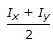B.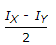C. IX + IY D.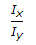Explanation:

No answer description available for this question. Let us discuss.

8.

Maximum shear stress theory for the failure of a material at the elastic limit, is known

 A. Guest's or Trecas' theory B. St. Venant's theory C. Rankine's theory D. Haig's theory E. Von Mises's theory.

Explanation:

No answer description available for this question. Let us discuss.

9.

The force in AC of the truss shown in the given figure, is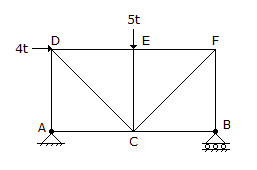A. 5t tension B. 4t tension C. 4t compression D. 5t compression E. None of these.

Explanation:

No answer description available for this question. Let us discuss.

10.

For determining the force in the member AB of the truss shown in the given figure by method of sections, the section is made to pass through AB, AD and ED and the moments are taken about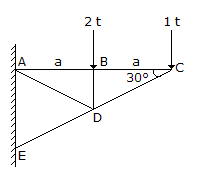A. joint C B. joint B C. joint D D. joint A